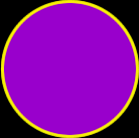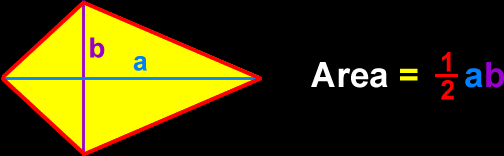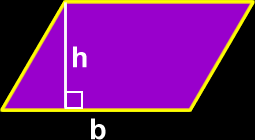# Areas

Formulas and instructions for finding the areas of circles, kites, parallelograms, rectangles, rhombi, squares, trapezoids and oddly shaped geometric objects
Just scroll down or click on what you want and I'll scroll down for you!

 area of a circle area of a kite area of a parallelogram area of a rectangle area of a rhombus area of a square area of a trapezoid area of an oddly shapedgeometric region

 The area of a circle:Area =where r = the radius of the circleand pi = 3.141592...The area of a kite: To find the area of a kite, multiply the lengths of the two diagonals and divide by 2 (same as multiplying by 1/2):The area of a parallelogram:To find the area of a parallelogram, just multiply the base length (b) times the height (h):Area = b x h Go here to see where this formula comes from!